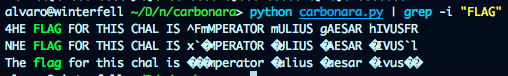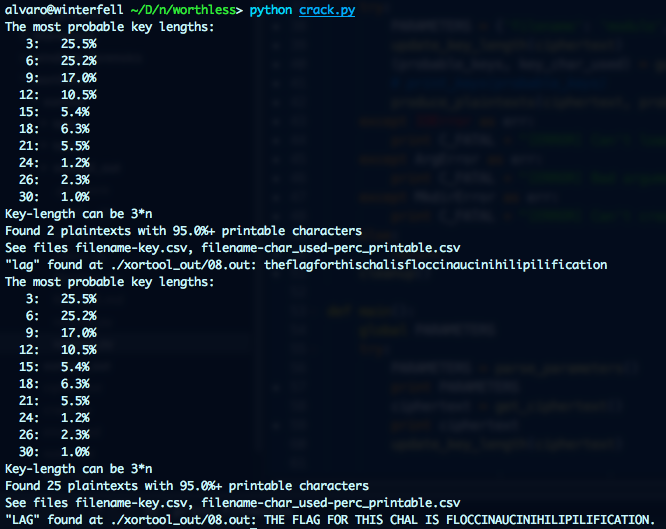# Carbonara

We are given the following ciphertext:

%96 7=28 [email protected] E9:D 492= :D iQx>[email protected] xF=:FD r26D2C s:GFDQ]

A simple shift shows interesting results:

ciphertext = "%96 7=28 [email protected] E9:D 492= :D iQx>[email protected] xF=:FD r26D2C s:GFDQ]"
size = len(ciphertext)
for i in range(0,100):
result=""
for c in ciphertext:
if ord(c) > 126 or ord(c) < 33:
result += c
else:
first = ord(c)+i
if first > 90:
first = 64 + (first - 90)
result += chr(first)
print(result)Here is were the history classes prove valuable, flag is:

Imperator Iulius Caesar Divus

# Worthless

We are given a bunch of 0’s and 1’s.

00010111000001110001010001100011 00001001000111010000001000001000 01110001000001010000000000000011
01100011000110110001100100001010 00011100011100010000000000000111 00010000000011110110111100011000
00010000011011110001011100001111 00000000000100100000000000000110 00011111000000100001101000010010
00001010000000010001100000001011 00000110000111010000101000011111 00011000000011110000011000010111
00001010000011000001000000010111 00000110000111100000110101100001


If we group them by bytes we get a 56 length binary. Our favorite xor key guessing tool: xortool by Hellman shows that a key of length 3n is possible. However it fails decrypting the message with “ ” (supposing it is a text) as the most frequent char. That is normal in such short texts. The idea to solve it is to pass all characters as most frequent chars for the analysis and then grep the results for words you may be expecting such “flag”.

from xortool.xortool import process
import os

def search(text):
rootdir = './xortool_out'
for subdir, dirs, files in os.walk(rootdir):
for file in files:
if ".out" in file:
f = open(os.path.join(subdir,file),'r')
if text in contents:
print "\"%s\" found at %s: %s" % (text, os.path.join(subdir,file), contents,)

original = "0001011100000111000101000110001100001001000111010000001000001000011100010000010100000000000000110110001100011011000110010000101000011100011100010000000000000111000100000000111101101111000110000001000001101111000101110000111100000000000100100000000000000110000111110000001000011010000100100000101000000001000110000000101100000110000111010000101000011111000110000000111100000110000101110000101000001100000100000001011100000110000111100000110101100001"
bytes = []
for i in range(0,len(original),8):
test = hex(int(original[i:i+8], 2))
bytes.append(test[2:].zfill(2))
ciphertext = ''.join(bytes).decode('hex')

# try lower letters as most frequest chars
process(ciphertext, [i for i in range(97,122)])
search("lag")

# try upper letters as most frequest chars
process(ciphertext, [i for i in range(65,90)])
search("LAG")


The result of running the script:Voila!